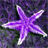# Raster statistics in script tool/Reclassify temp rasters

2229
0
05-16-2013 10:01 PMOccasional Contributor
Hi,

First off, apologies if this is not the correct forum for this question....

I have a Python script tool that iterates through a polygon feature class and extracts from a DEM to perform some analysis. As part of this workflow, I need to reclassify the raster subset using RemapRange. This works ok in the Python window, but the script tool returns:

ERROR 010423: Slope_Extrac1.RASTER.1(Band_1) does not have valid statistics as required by the operation.
Failed to execute (Reclassify).

I presume that this is because I am using Python to store the temp rasters:
```# Slope
slope = Slope(demExtract, "DEGREE","1")

# Set up classes
remapSlope = RemapRange([[0, 3, 1],
[3, 7, 2],
[7, 15, 3],
[15, 20, 4],
[20, 25, 5],
[25, 35, 6],
[35, 90, 7]])
# Reclass slope
slopeReclass = Reclassify(slope,"Value",remapSlope)```

If I try to calculate statistics for the variable 'slope', I get:

ERROR 999999: Error executing function.
The workspace is not connected.
Failed to execute (CalculateStatistics).

(even though I have set up arcpy.env.workspace and arcpy.env.scratchWorkspace).

I could write the temp rasters to disk (and run the calculate statistics tool, if required), but doing this several hundred times might affect performance.

I could also reclass the original DEM in the example above, but at some point in the workflow quantiles or standard deviation will be used to generate the RemapRange values. (Somes ideas on that would be welcome, too.)

So my question (finally) is: what is the most efficient way to reclassify several hundred temporary rasters?

Thanks,

Matt
Tags (3)
0 Replies